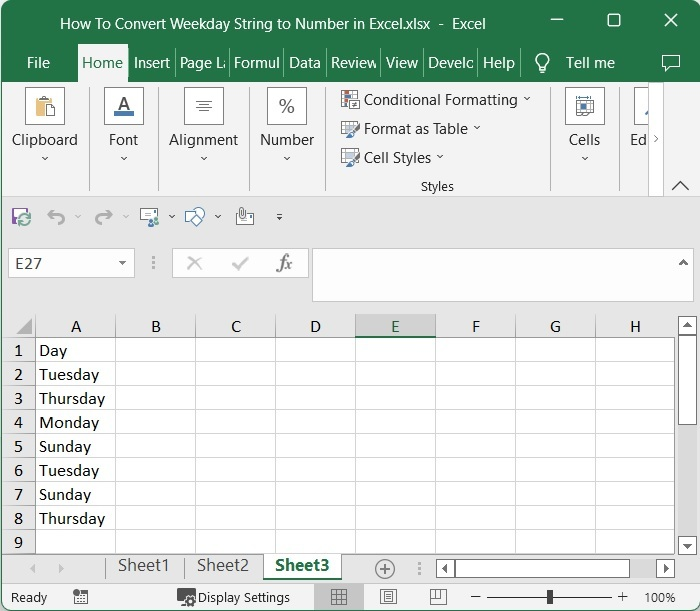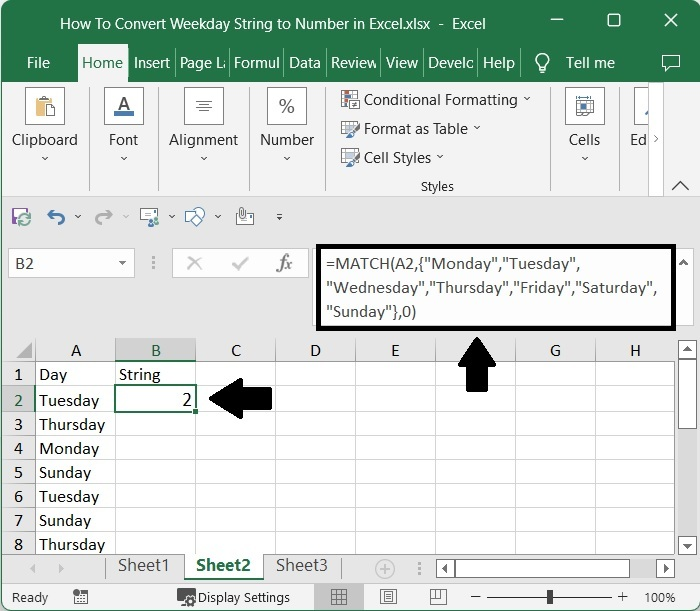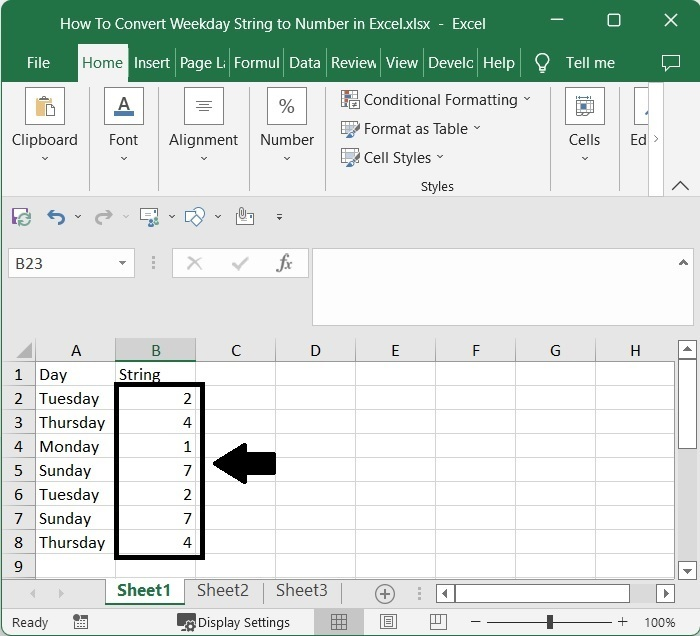# How to Convert Weekday String to Number in Excel?

Sometimes in Excel, you would need to convert the given day name to a number, like one for Monday, two for Tuesday, and so on. If we try to convert the days manually, we can use it if there are fewer values; when there are more values, we can’t use the manual process as it can be a time-consuming and inaccurate process. We can convert the days to numbers using the formulas to save a lot of time when performing the task.

Read this tutorial to learn how you can convert a weekday string to a number in Excel. Even though there is no direct formula available, we can create one using simple techniques. We can complete this task using the match function as described in this tutorial.

## Converting Weekday String to Number in Excel

Here, we will first get any result using the MATCH formula, then get all the results using the auto-fill handle. Let's go over a simple procedure for converting a weekday string to a number in Excel using formulas. Our approach will use the help of the MATCH function to complete the task.

### Step 1

Consider the following image of an excel sheet with data that is a list of days.Now, in cell B2, click on an empty cell and enter the formula as follows −

=MATCH(A2,"Monday","Tuesday","Wednesday","Thursday","Friday","Saturday","Sunday",0)


And press enter to get our first result, which looks like the image below. In the formula, A2 is the address of the cell where our value is present on the sheet.

Empty cell > Formula > Enter### Step 2

Then drag down from the first result using the auto-fill handle to get all the results, and our final output will be similar to the below image.## Conclusion

In this tutorial, we have used a simple example to demonstrate how you can convert a weekday string to a number in Excel.